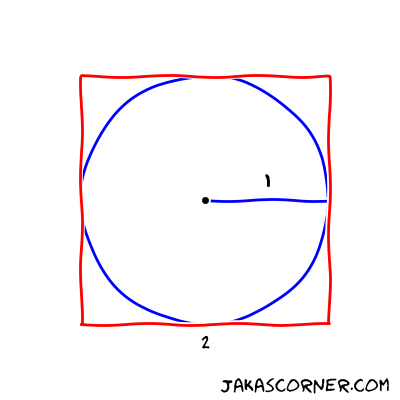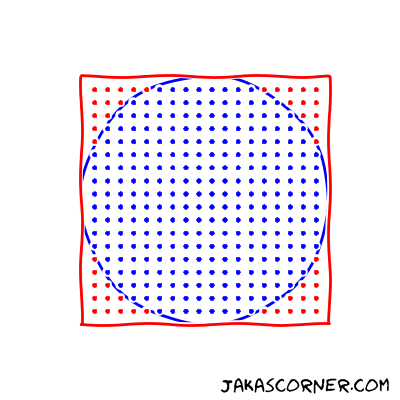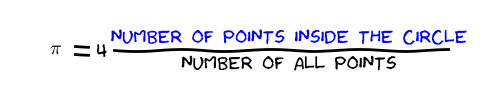The Monte Carlo methods are a class of algorithms that rely on repeated random sampling to calculate the result. These methods are used in Engineering, Statistics, Finance and many other fields.

Our objective is to familiarize ourselves with a Monte Carlo method for computing the Pi (`π`) number. First, we will implement a sequential version of the method. And then, we will use OpenMP to parallelize the sequential version.

## Computation of Pi

If you don’t know what `π` is, it is that number which has something to do with circles. More precisely, the area of the disk with the radius `r` is equal to `π * r^2`.If the radius of the circle is equal to one, then the area of the disk is equal to `π`.

Further, let’s inscribe a circle in a square. If the length of the side of the square is equal to two, then the radius of the inscribed circle is equal to one.Now, we divide the area of the circle with the area of the squareIf we multiply this fraction by four, we get the value of `π`.

A simple way to compute the fraction is to generate the points in the square and count the number of points which lie in the inside of the circle.The approximation of the `π` is# Monte Carlo method for Pi

The Monte Carlo method uses the previous idea to compute the value of `π`. The method randomly generates points in the square `[-1, 1] x [-1, 1]` and counts the number of points in the inside of the unit circle. The result is four times the fraction between the number of points which lie in the inside of the circle and the number of all generated points.

# Uniform points

The first step of the implementation is to uniformly generate random points. The `<random>` standard library is appropriate for such a task. In order to generate random points with the library, we need to specify two things:

• the generator, which generates the uniformly distributed numbers and
• the distribution, which transforms the numbers from the generator into the numbers that follow a specific random distribution.

We use `std::default_random_engine` as the generator and `std::uniform_real_distribution<double>` as the distribution. We initialize the internal state of the generator with the `std::default_random_engine::seed` member function. Finally, we generate an uniform random sample ```(x, y)``` in `[-1, 1] x [-1, 1]`.

We encapsulate the random generation of the points in the class `UniformDistribution`. The constructor takes care for the initialization of the generator and the distribution. The member function `UniformDistribution::sample` generates a random sample in the interval `[-1, 1]`. The following code is then equivalent to the code above.

The source code of the `UniformDistribution` is available here.

# Monte Carlo method

The next function describes the Monte Carlo algorithm for `π`.

The input of the function is the number of samples. The function generates samples `(x, y)` in `[-1, 1] x [-1, 1]` and counts all the samples which lie in the inside of the circle with radius one. The approximation of the `π` is four times the fraction between the number of samples, which lie in the inside of the circle, and the total number of samples. This approximation formula for the value of `π` was derived above.

## Examples

The quality of the result depends on the total number of samples. The higher number of samples increases the accuracy of the solution. The next output illustrates this phenomenon.

We can see that the results are more accurate with higher number of samples. Another observation is that the Monte Carlo method is an approximation algorithm – it returns an approximation of the result not the exact solution.

Since the algorithm depends on the random sapling, two executions of the same program give different results.

A valid concern is whether the Monte Carlo methods are useful at all. Why would someone use the method which returns approximate results (and these results might vary from one execution to the other)? Of course the Monte Carlo method for `π` is not very useful, because the value of `π` is very well known.

But there exist problems for which it is impossible to compute results in a reasonable time. Here, the Monte Carlo methods are useful, because they can provide an approximation of the result within an acceptable time. Sometimes, it is better to have an approximation of the result than no result.

Since the Monte Carlo methods depend on a repeated independent random sampling, they are especially appropriate for parallel execution. Each thread can independently compute many samples.

In the next article, we will parallelize the Monte Carlo method for `π` with the help of OpenMP.

## Summary

In this article, we learned a Monte Carlo method for the approximation of `π`.